﻿ 小学数学二年级上册乘法算式的意义 - 小学数学 - 智慧山

# 小学数学二年级上册乘法算式的意义

2019-01-02 10:15:00

6152

乘法算式的意义、写法和读法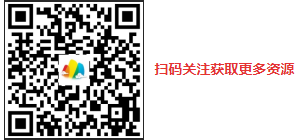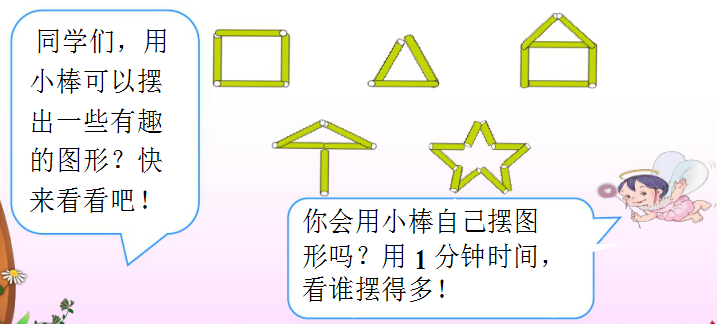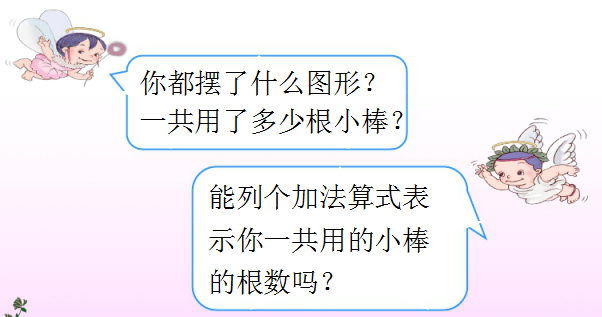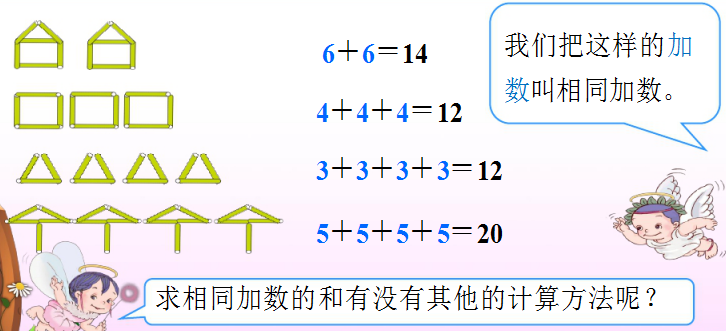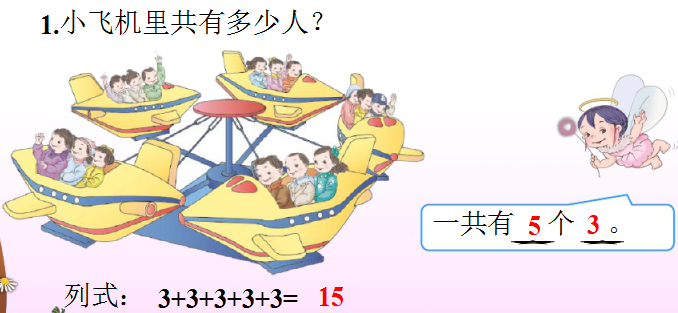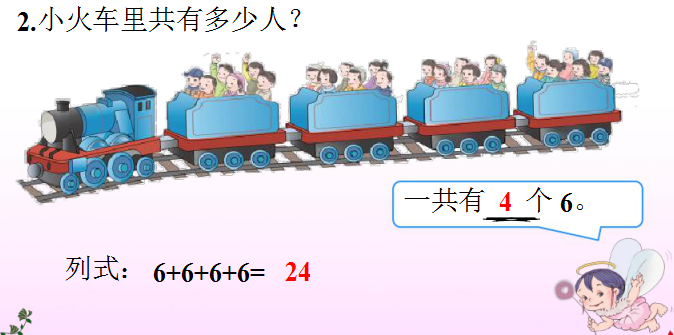（1 3+3+3+3+3=15

5 ）个（   3

2 6+6+6+6=24

4 ）个（  6

3 2+2+2+2+2+2+2=14

7 ）个（  2

1 3+3+3+3+3=15   5 ）个（  3

5×3=15 读作:5×3等于15

2 6+6+6+6=24   4 ）个（  6

3 2+2+2+2+2+2+2=14  7  ）个（2

7 ×（ 2 = 14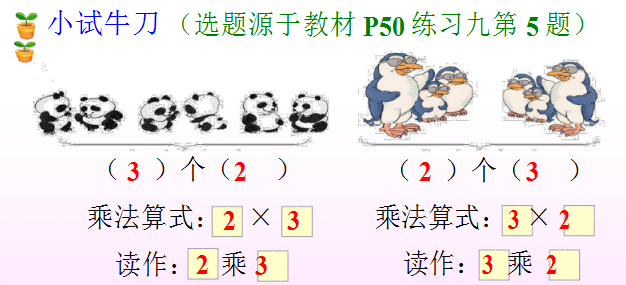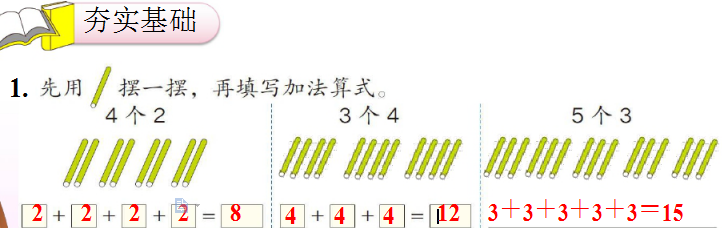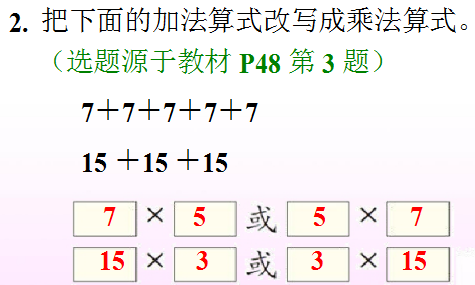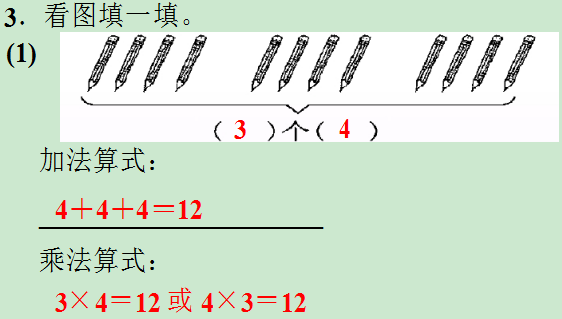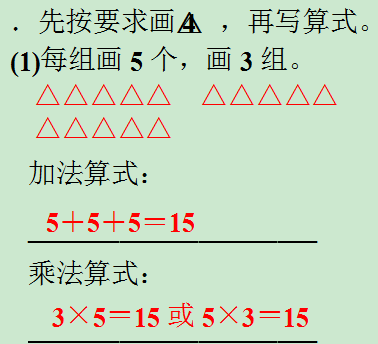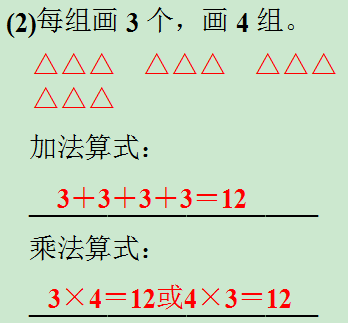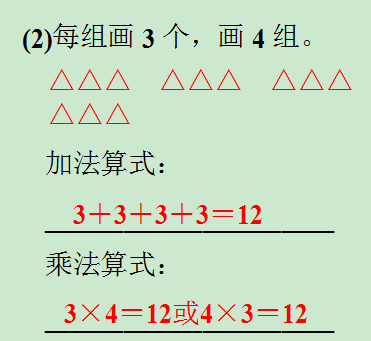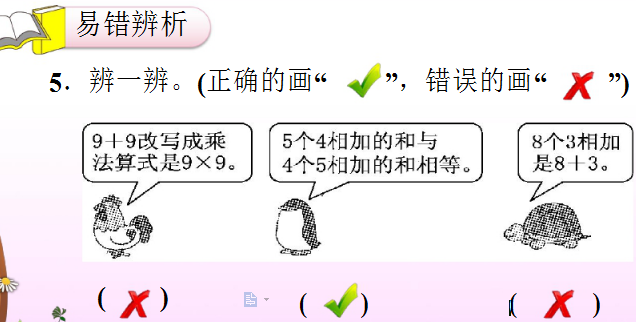6.  星期天，爸爸、妈妈带明明一起去超市买蛋

4+4+412（个）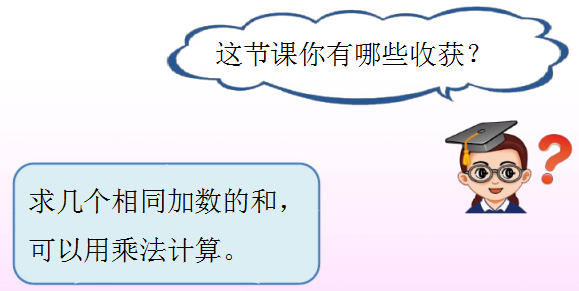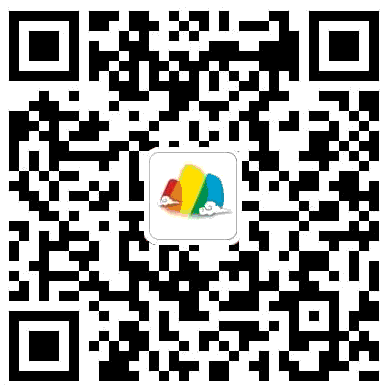发表评论
评论通过审核之后才会显示。
分类免费预约体验课